6 Oct 2015

# My background

• I am a quantitative psychologist interested in integrating meta-analysis into the structural equation modeling (SEM) framework.
• I am co-editing (with Adam Hafdahl) a special issue on meta-analytic structural equation modeling that will appear in Research Synthesis Methods (hopefully appear in Issue 2 2016).
• I am co-editing (with Michael Bosnjak and Wolfgang Viechtbauer) a book series on SpringerBriefs in Systematic Reviews and Meta-Analysis published by Springer. The first book will be on the topic of meta-analytic structural equation modeling (MASEM) written by Suzanne Jak.
• Besides this line of research, I am also interested in the following topics: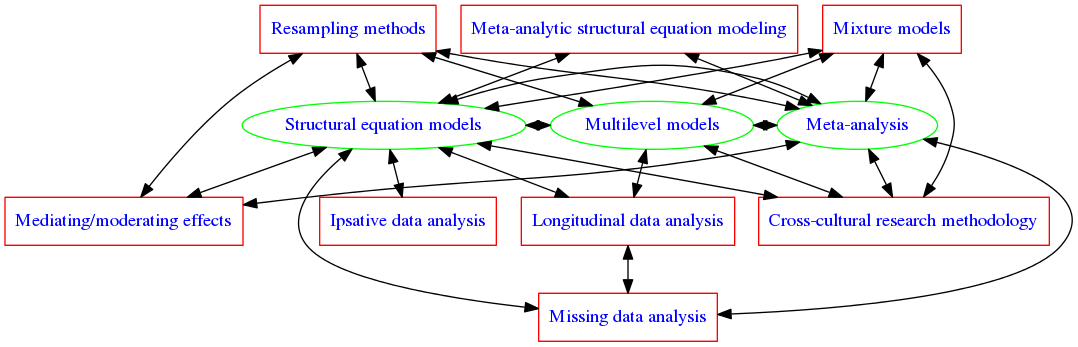# Types of data analyses

• Primary data analysis: Data are collected and analyzed for specific research questions.
• Secondary data analysis: Existing data sets are re-analyzed to address research questions that are different from the original ones.
• Meta-analysis: Published studies are extracted and combined to draw general conclusions.

# Very brief history of meta-analysis (1)

• Karl Pearson (1904) was probably the first one applying meta-analytic ideas to combine correlations between inoculation for typhoid fever and mortality from independent samples.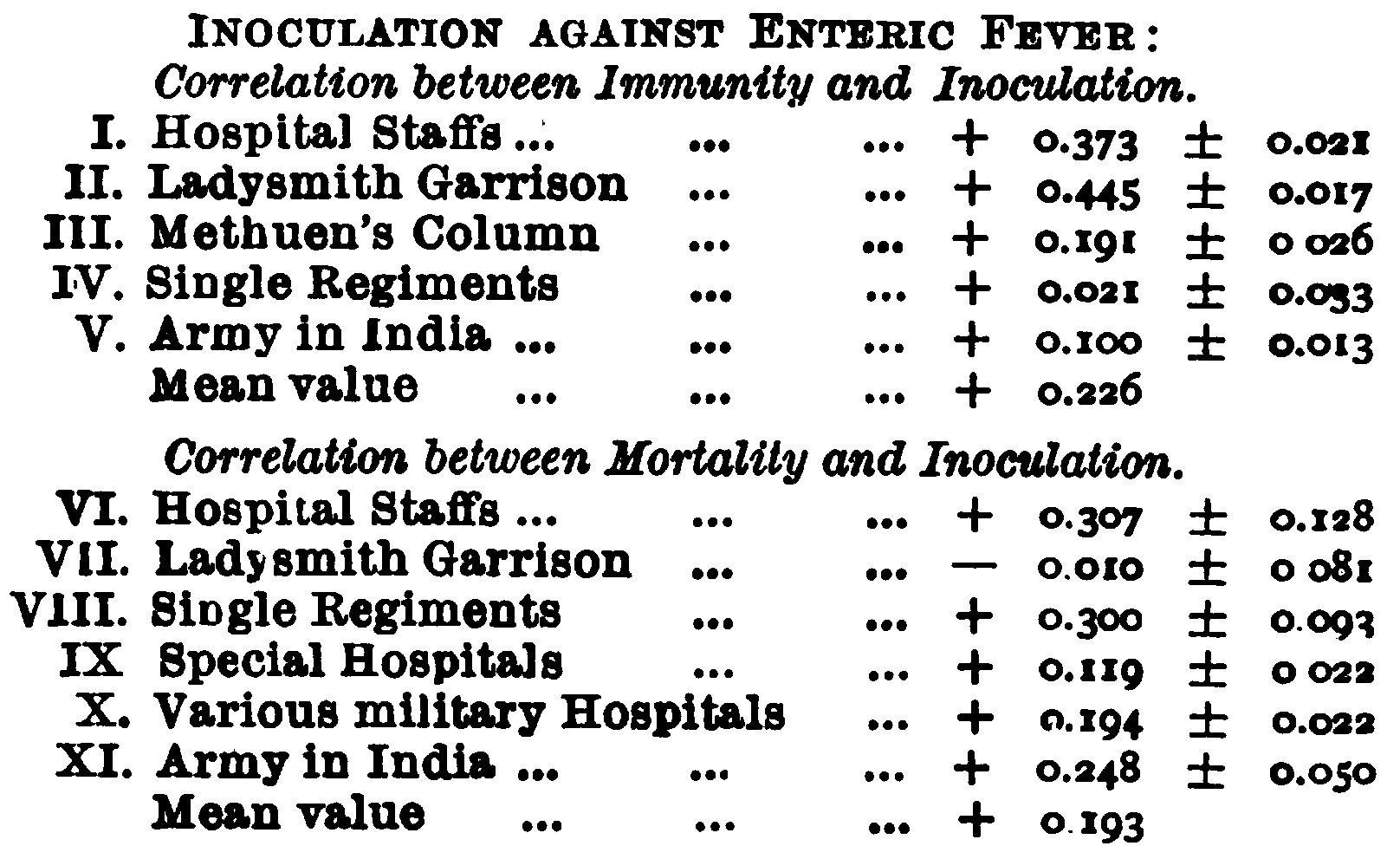Pearson, K. (1904). Report on certain enteric fever inoculation statistics. BMJ, 2(2288), 1243-1246.

# Very brief history of meta-analysis (2)

• The term meta-analysis was coined by Gene V. Glass (1976) in educational research: “the statistical analysis of a large collection of analysis results from individual studies for the purpose of integrating the findings” (Glass, 1976, p.3).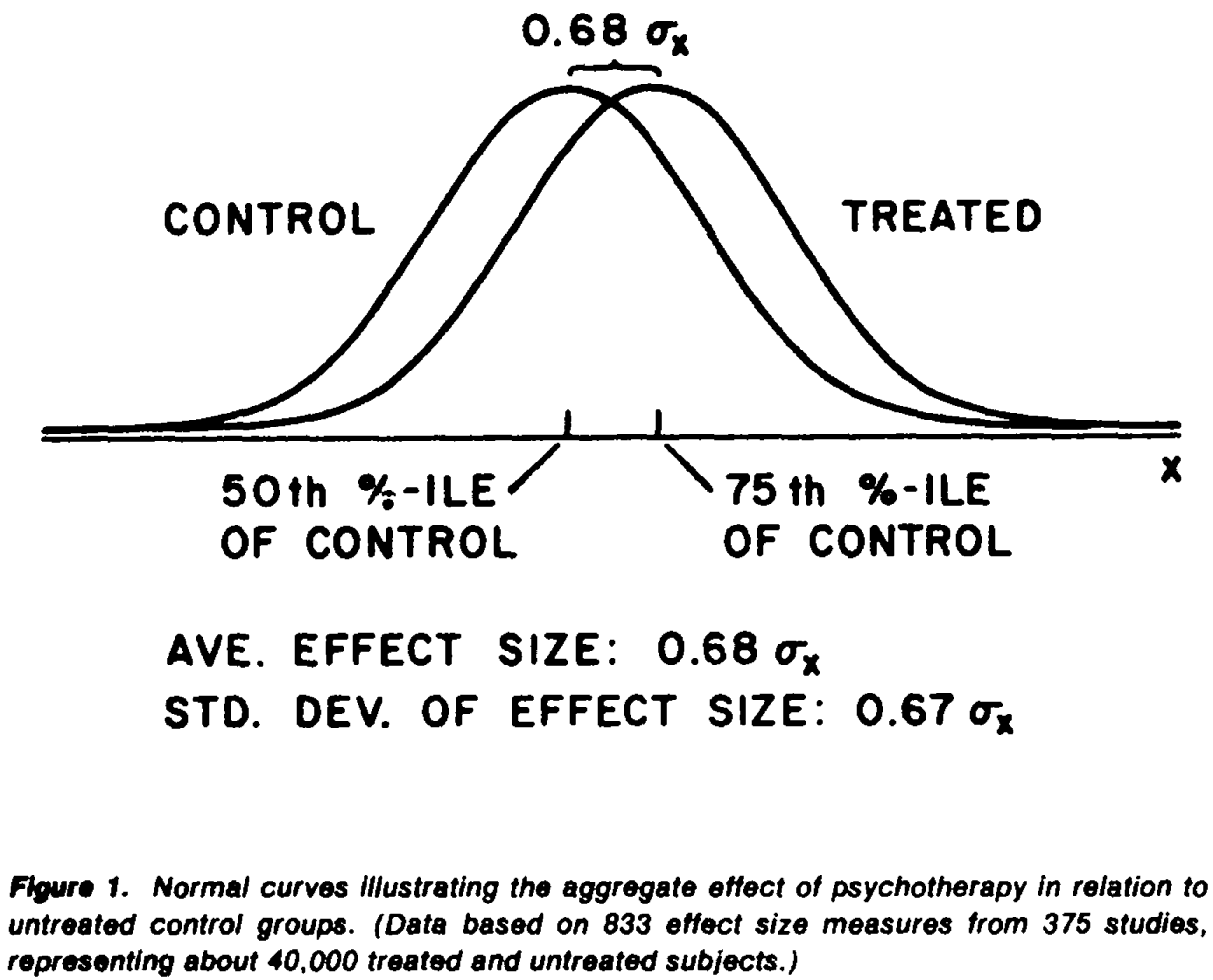• It became popular in social and behavioral sciences and then in medical science.

Glass, G. V. (1976). Primary, secondary, and meta-analysis of research. Educational Researcher, 5(10), 3-8.

# Very brief history of meta-analysis (3)

• It is known as validity generalization in Industrial and Organizational Psychology (Schmidt & Hunter, 2015).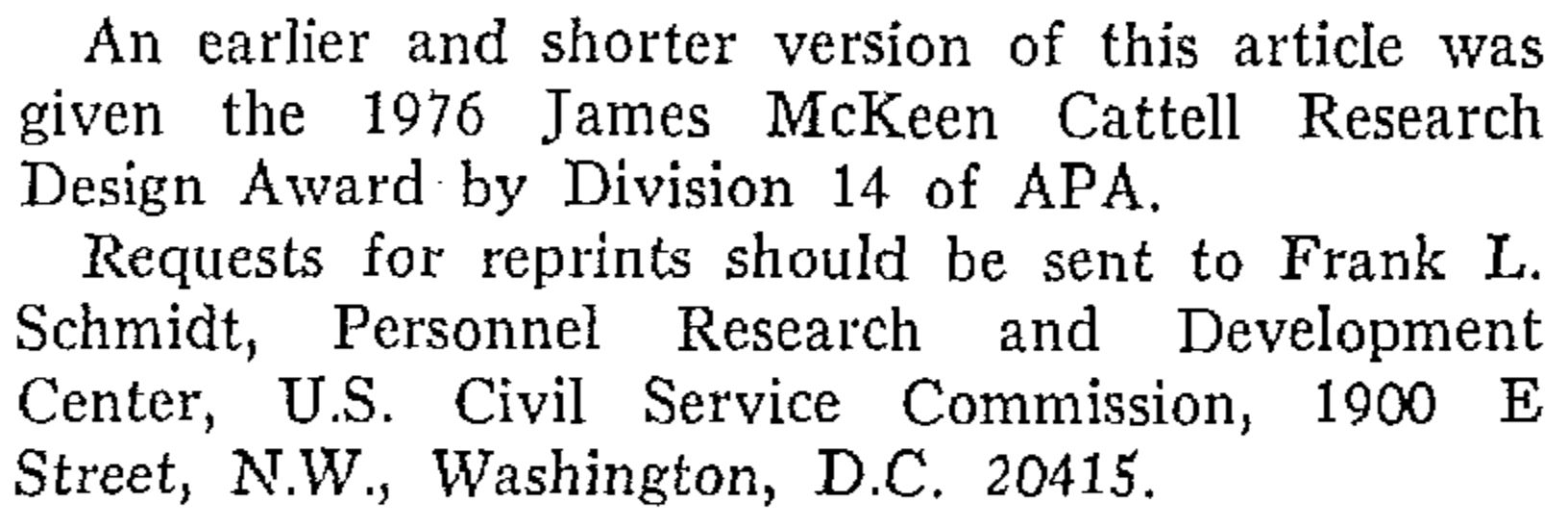• “Unaware of Glass’s work, we developed our meta-analysis methods in 1975… we won the Cattell award for 1976, but the 1-year delay in publication (Schmidt & Hunter, 1977) meant that our first meta-analysis article appeared 1 year after Glass’s” (Schmidt & Hunter, 2015, xxvi).

Schmidt, F. L., & Hunter, J. E. (1977). Development of a general solution to the problem of validity generalization. Journal of Applied Psychology, 62(5), 529-540.

Schmidt, F. L., & Hunter, J. E. (2015). Methods of meta-analysis: Correcting error and bias in research findings (3rd ed.). Thousand Oaks, CA: Sage.

# Statistical models in meta-analysis

• Univariate meta-analysis: fixed-, random-, and mixed-effects models
• Multivariate and three-level meta-analyses
• Network meta-analysis
• Individual patient data meta-analysis
• Meta-analytic structural equation modeling

# SEM

• SEM is a flexible modeling technique to test hypothesized models.
• The proposed models can be specified as either path diagrams, equations, or matrices.
• It integrates several statistical techniques into a single framework–path analysis in biology and sociology, factor analysis in psychology, and simultaneous equations in economics.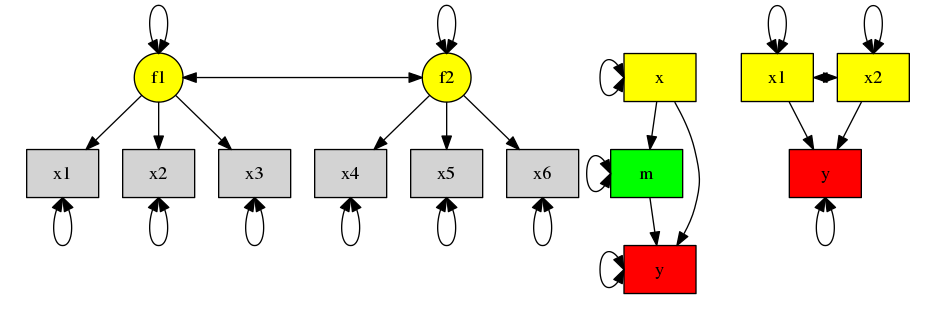# Statistical models in SEM

• General (and generalized) linear models, e.g., regression, ANOVA to MANOVA
• Confirmatory factor analysis (CFA), SEM, latent growth model
• Item response theory (IRT)
• Multilevel modeling
• Analysis of binary data, missing data, non-normal data, and mixture modeling
• Meta-analysis and meta-analytic structural equation modeling

# Why do we (I) want to integrate these two lines of research?

• These two techniques were treated as unrelated topics in the literature. They have different terminologies, software, and even journals (Research Synthesis Methods, and Structural Equation Modeling: A Multidisciplinary Journal).
• There is limited communication between these two groups of researchers.
• Advances in one area has limited influence on the other area.
• Integrating meta-analysis into SEM helps to develop a unified statistical approach.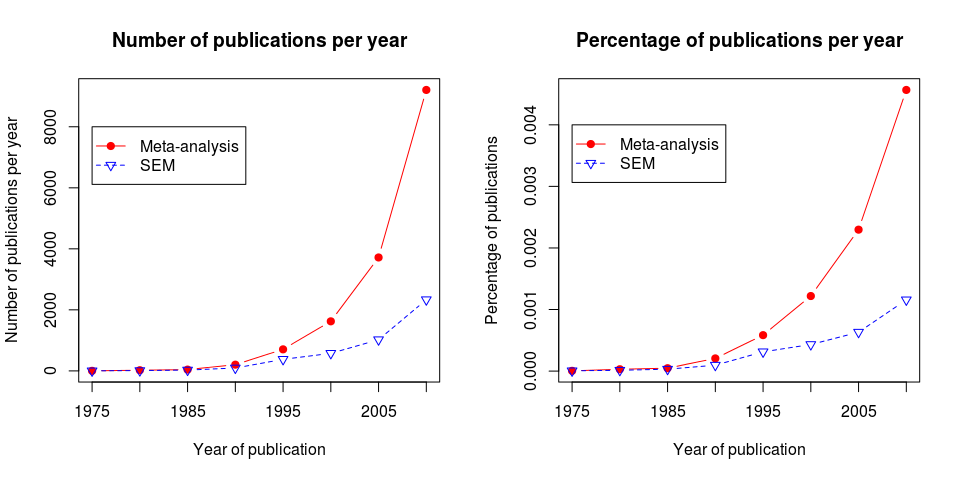# Are meta-analyses structural equation models?

• There is a one-to-one correspondence between a meta-analytic model and a structural equation model.
Meta-analysis SEM
Study (k study) Subject (N subjects)
Observed (sample) effect sizes Observed scores
True (population) effect sizes Latent scores
Average effect Mean of latent scores
Heterogeneity variance Variance of latent scores
Sampling error Measurement error
Moderator Predictor

# What are the advantages of the SEM-based meta-analysis?

• The true effect sizes are conceptualized as latent variables in SEM. The latent variables can used as dependent or independent variables.
• Likelihood-based confidence intervals (LBCI) may be constructed for the parameters or functions of parameters, e.g., $$I^2$$.
• Missing moderators can be handled with full information maximum likelihood (FIML).
• Constraints can be easily imposed in the models.

# Effect sizes

• Effect sizes ($$y_i$$) and their sampling variances ($$v_i$$) are the key ingredients in meta-analysis.
• Important properties of effect sizes for a meta-analysis:
• Directional, e.g., $$R^2$$, $$\eta^2$$, and $$\omega^2$$ are usually not recommended;
• Relatively independent of sample size;
• Asymptotically unbiased; and
• Approximately normally distributed.
• We assume that the sampling variances are known in a meta-analysis:
• $$y_i = f_i + e_i$$,
• where $$f_i$$ is the true effect size and $$v_i=Var(e_i)$$ is the known sampling variance.
• Transformations may be required to stabilize the sampling variances of some effect sizes, e.g., log of odds ratio and Fisher’s z transformed score of correlation coefficient.

# Common effect sizes and their sampling variances

• Here are some common effect sizes (Cheung et al. 2012).
• The metafor package (Viechtbauer, 2010) provides functions to calculate typical effect sizes and their sampling variances.